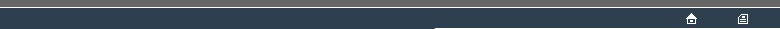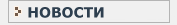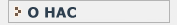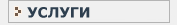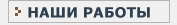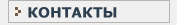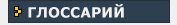СТАТИСТИЧЕСКИЙ В данном разделе Вы найдете все интересующие Вас термины и определения, связанные с статистикой и статистическими исследованиями.КЛИНИЧЕСКИЕ ИССЛЕДОВАНИЯ Необходимый перечень терминов и определений, используемых при проведении клинических испытанийF FACTORIAL The FACTORIAL operator is applicable to a non-negative integer quantity. It is notated as the postfixed symbol '!'. The resulting value is the product of the increasing integer values from 1 up to the value of the argument quantity. For instance : 3! is 1x2x3 = 6. By convention 0! is taken as producing the value 1. FACTORIAL values increase very rapidly wityh increase in the argument value; this rapid growth is represented in the similarly rapid growth in numbers of COMBINATIONS. FISHER TEST(1) [Named after the statistician RA Fisher()]. This is an EXACT TEST(1) to examine whether the pattern of counts in a 2x2 cross classification departs from expectations based upon the marginal totals for the rows and columns. Such a test is useful to examine difference in rate between two binomial outcomes. The RANDOMISATION SET consists of those reassignments of the units which produce tables with the same row- and column- totals as the OUTCOME. The RANDOMISATION SET will thus consist of a number of tables with different respective patterns of counts; each such table will have a number of possible RANDOMISATIONS which may be a very large number. For this test there are several reasonable TEST STATISTICs, including : the count in any one of the 4 cells, CHI-SQUARED(1), or the number of RANDOMISATIONS for each 2x2 table with the given row- and column- totals; these are EQUIVALENT TEST STATISTICS. The calculation for the FISHER TEST(1) is relatively undemanding computationally, making reference to the algebra of the hypergeometric distribution, and the test was widely used before the appearance of COMPUTERs. This test has historically been regarded as superior to the use of CHI-SQUARED(2) where sample sizes are small. Statistical tables have been published for the FISHER TEST(1) for a number of small 2x2 tables defined in terms of row- and column- totals. Also see FISHER TEST(2), TWO-WAY TABLE. FISHER TEST(2) [()] This is also known as the FREEMAN-HALTON TEST. It is an extension of the logic of the FISHER TEST(1), for a 2-way classification of counts where the extent of the cross-classification may be greater than 2x2. The RANDOMISATION SET for an EXHAUSTIVE RANDOMISATION TEST (EXACT TEST(1)) can be defined in the same way as for the FISHER TEST(1). However, the various TEST STATISTICs applicable when considering the FISHER TEST(1) will not all be definable and will not clearly be EQUIVALENT TEST STATISTICs. The TEST STATISTIC which is used is the number of RE-RANDOMISATIONS for each table with the given row- and column- totals; this TEST STATISTIC has the drawback of lacking any descriptive significance in terms of the EXPERIMENTAL DESIGN. FORTRAN [Name is an acronym : FORmula TRANslator]. A very long established and widely implemented PROGRAMMING LANGUAGE, specialised substantially for numerical applications. A number of STANDARD PROGRAMMING LANGUAGE versions of FORTRAN have established at various dates (e.g. FORTRAN IV, FORTRAN 90), approved as standard by ANSI and ISO. FREEMAN-HALTON TEST See FISHER TEST(2).F
FACTORIAL
FISHER TEST(1)
FISHER TEST(2)
FORTRAN
FREEMAN-HALTON TESTНовости   О нас   Услуги   Наши работы   Статьи   Контакты   Глоссарий
Статистическая помощь! © 2005 - 2022 • Защита авторских прав